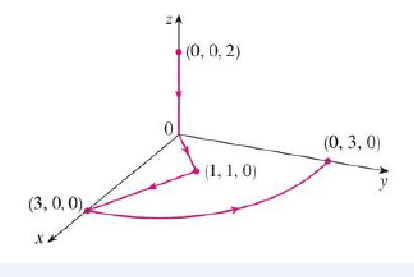Chapter 16, Problem 37RE

Chapter
Section
Textbook Problem

Let F(x, y, z) = (3x2 yz – 3y) i + (x3z – 3x) j + (x3y + 2z) kEvaluate ∫C F • dr, where C is the curve with initial point (0, 0, 2) and terminal point (0, 3, 0) shown in the figure.To determine

To evaluate: The value of CFdr , where C is the curve with initial point (0,0,2) and terminal point (0,3,0) .

Explanation

Given data:

F(x,y,z)=(3x2yz3y)i+(x3z3x)j+(x3y+2z)k (1)

Formula used:

Consider the standard equation of an curl F for F=Pi+Qj+Rk

curlF=|ijkxyzPQR| (2)

Find the value of curlF .

Substitute (3x2yz3y) for P , (x3z3x) for Q and (x3y+2z) for R in equation (2),

curlF=|ijkxyz(3x2yz3y)(x3z3x)(x3y+2z)|={[y(x3y+2z)z(x3z3x)]i[x(x3y+2z)z(3x2yz3y)]j+[x(x3z3x)y(3x2yz3y)]k}=[x3x3]i[3x2y3x2y]j+[3x2z3x2z]k=0

Since curlF=0 , the F is a conservative vector and the domain of F is 3 .

Consider f=fx(x,y,z)i+fy(x,y,z)j+fz(x,y,z)k .

Write the relation between the potential function f and vector field F .

F=f

Substitute fx(x,y,z)i+fy(x,y,z)j+fz(x,y,z)k for f ,

F=fx(x,y,z)i+fy(x,y,z)j+fz(x,y,z)k (3)

Compare the equation (3) and equation (1).

fx(x,y,z)=3x2yz3y (4)

fy(x,y,z)=x3z3x (5)

fz(x,y,z)=x3y+2z (6)

Integrate equation (4) with respect to x.

f(x,y,z)=(3x2yz3y)dx=3x33yz3xy+g(y,z)

f(x,y,z)=x3yz3xy+g(y,z) (7)

Apply partial differentiation with respect to y on both sides of equation (7).

fy(x,y,z)=y[x3yz3xy+g(y,z)]=y(x3yz)y(3xy)+y[g(y,z)]=(x3z)y(y)3xy(y)+y[g(y,z)]

fy(x,y,z)=x3z3x+g(y,z)

Consider the value of g(y,z)=h(z)

Still sussing out bartleby?

Check out a sample textbook solution.

See a sample solution

The Solution to Your Study Problems

Bartleby provides explanations to thousands of textbook problems written by our experts, many with advanced degrees!

Get Started

Factoring Common Factor Factor out the common factor. 64. 3x4 6x3 x2

Precalculus: Mathematics for Calculus (Standalone Book)

Solve the equations in Exercises 126. (x21)2(x+2)3(x21)3(x+2)2=0

Finite Mathematics and Applied Calculus (MindTap Course List)

solve the equation by using the quadratic formula. 139. 2x2 + 4x 3 = 0

Applied Calculus for the Managerial, Life, and Social Sciences: A Brief Approach

= _____. 0 does not exist

Study Guide for Stewart's Single Variable Calculus: Early Transcendentals, 8th

The polar form for the graph at the right is:

Study Guide for Stewart's Multivariable Calculus, 8th